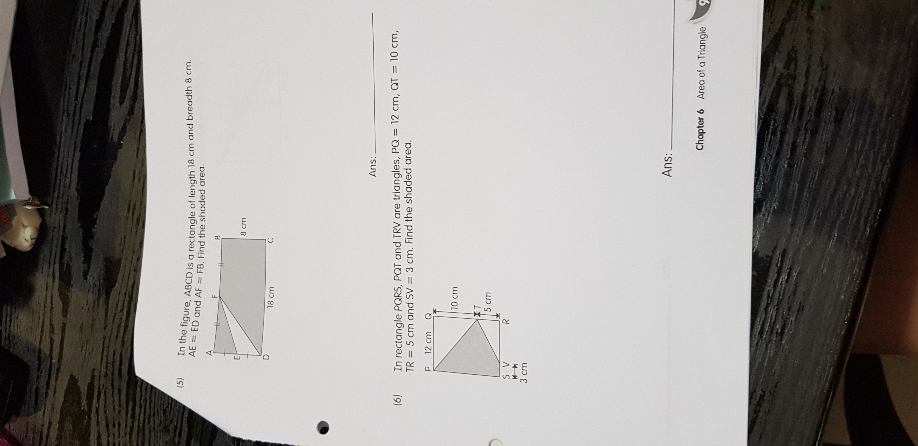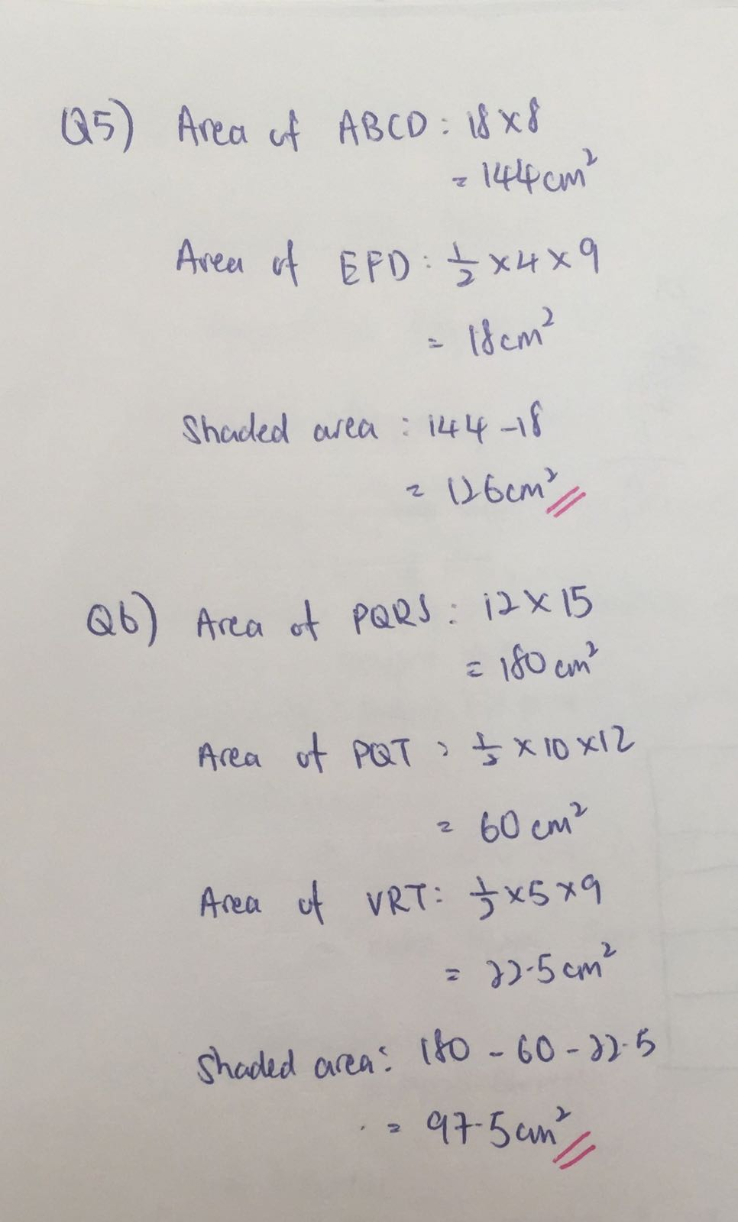# QuestionCan help me solve? Thanks

Source: Geylang Methodist School

(5)
18 x 8 = 144
8/2 = 4
18/2 = 9
(1/2) x 4 x 9 = 18
144 – 18 = 126

Ans : 126 sq cm.

(6)
Drawing a line from point S to point T to obtain 2 triangles, PST and STV,
the shaded area = (1/2) x (10 + 5) x 12 + (1/2) x 3 x 5 = 97.5

Ans : 97.5 sq cm

0 Replies 0 Likes